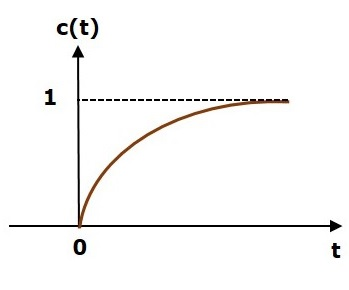# Control Systems – Stability

Stability is an important concept. In this chapter, let us discuss the stability of system and types of systems based on stability.

## What is Stability?

A system is said to be stable, if its output is under control. Otherwise, it is said to be unstable. A stable system produces a bounded output for a given bounded input.

The following figure shows the response of a stable system.This is the response of first order control system for unit step input. This response has the values between 0 and 1. So, it is bounded output. We know that the unit step signal has the value of one for all positive values of t including zero. So, it is bounded input. Therefore, the first order control system is stable since both the input and the output are bounded.

## Types of Systems based on Stability

We can classify the systems based on stability as follows.

• Absolutely stable system
• Conditionally stable system
• Marginally stable system

### Absolutely Stable System

If the system is stable for all the range of system component values, then it is known as the absolutely stable system. The open loop control system is absolutely stable if all the poles of the open loop transfer function present in left half of âs` plane. Similarly, the closed loop control system is absolutely stable if all the poles of the closed loop transfer function present in the left half of the âs` plane.

### Conditionally Stable System

If the system is stable for a certain range of system component values, then it is known as conditionally stable system.

### Marginally Stable System

If the system is stable by producing an output signal with constant amplitude and constant frequency of oscillations for bounded input, then it is known as marginally stable system. The open loop control system is marginally stable if any two poles of the open loop transfer function is present on the imaginary axis. Similarly, the closed loop control system is marginally stable if any two poles of the closed loop transfer function is present on the imaginary axis.